Write-up for hxp's tetres2019 challenge
29.12.2019

### The game

The game is tetris where, according to the rules, one has to score a lot of points to receive the flag.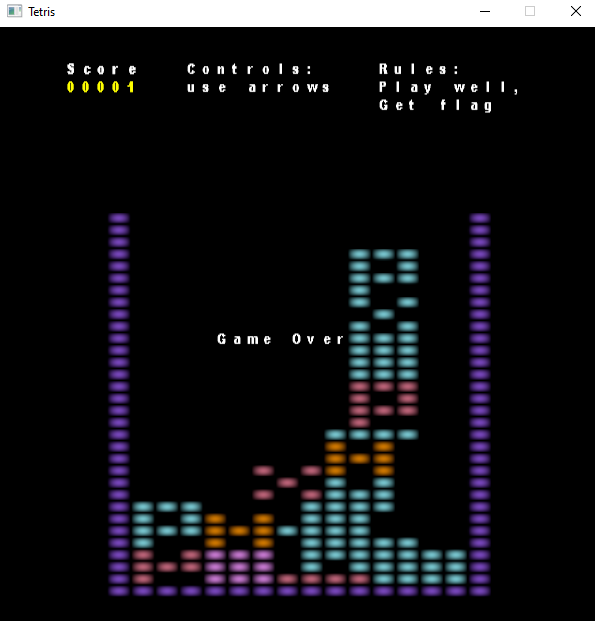The game doesn't really give good figures to be able to score easily, the target score is not disclosed and since this is a reverse engineering challenge, it's probably be best to go in the direction of reversing the game.

The challenge explicitly mentions that OpenGL 4.6 is required and that it only works on NVIDIA and Intel GPUs, so it would be best to look at the game in a graphics debugger.

### Graphics debugger

I used RenderDoc as a graphics debugger to see what OpenGL calls are made and what the main rendering loop looks like.We can see that there are only two GL calls which make something useful: glDispatchCompute and glDrawArrays. glDispatchCompute schedules a compute program to be executed and glDrawArrays schedules some primitives to be rendered.

We can also view resources in the game like textures, shaders and the whole GL state.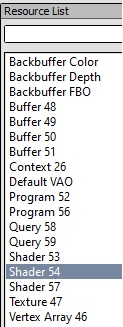By examining the compute shader, we can deduce that all of the game logic happens in it.

``````layout(set = 0, binding = 0, std430) coherent buffer State
{
int arr;
} _35;

layout(set = 0, binding = 1, std430) coherent buffer State2
{
int arr2;
} _234;

layout(set = 0, binding = 3, std430) buffer MyBlock
{
int x;
int y;
int tetris;
int dirx;
int diry;
int tock;
int mhm;
int idid;
} _279;

layout(set = 0, binding = 2, std430) coherent buffer Meta
{
int gen;
int abra;
int chch;
int chacha;
uint d;
} _299;
``````

The _35, _234, _279 and _299 buffers contain state information and are used throughout the whole program. In particular, the Meta::d buffer is used in the following snippet where it looks like something is being decoded:

``````if ((uint(lowest) == row) && (row != 0u))
{
uint q = _299.d[(col + uint(_299.abra)) % 32u];
q ^= (uint(_299.abra) + col);
_299.d[(col + uint(_299.abra)) % 32u] = (q << uint(25)) | (q >> uint(10));
q = _299.d[((col + uint(_299.abra)) + 16u) % 32u];
q ^= ((uint(_299.abra) + col) + 16u);
_299.d[((col + uint(_299.abra)) + 16u) % 32u] = (q << uint(25)) | (q >> uint(10));
}
``````

And the _299.abra variable keeps the score and is being incremented in a previous location in code:

``````if ((uint(lowest) == row) && (row != 0u))
{
if ((col != 0u) && (col != 15u))
{
for (int r = int(row); r < 32; r++)
{
_234.arr2[(r * 16) + int(col)] = _35.arr[((r + 1) * 16) + int(col)];
_35.arr[(r * 16) + int(col)] = _35.arr[((r + 1) * 16) + int(col)];
}
}
if (col == 0u)
{
_299.abra++;
}
}
}
``````

We can also see what GL calls were used to create the compute shader which will become important later: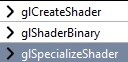glShaderBinary loads a binary file in a particular format and in this case it is in the SPIR-V format which was originally created to be used for the Vulkan API. glSpecializeShader is used to change constants and specify an entry point. For this shader it only specifies the main() entry point.

Other than that there isn't much else interesting in the compute shader. Everything else is logic for moving blocks and collision detection.

Next we examine the vertex and fragment shader used for rendering. The vertex shader doesn't do much but generate four vertices to create a rectangle to render to. This is a standard hacky way for creating a surface to render onto. The fragment shader is fairly large but contains a lot of repetitive code. The same GPU buffers persist in the fragment shader which means that they are shared with the compute shader. There are a lot of arrays with indices which are used to compute coordinates in a texture.

The arrays:

``````int msg = { 86, 56, 71, 37, 51 };
int msg_hoho = {
get_num(s, 10000), get_num(s, 1000), get_num(s, 100), get_num(s, 10), get_num(s, 1) };
int over = { 93, 83, 59, 51, 0, 72, 53, 51, 37 };
int controls = { 95, 71, 91, 49, 37, 71, 44, 75, 40 };
int controls_cmd = { 25, 75, 51, 0, 83, 37, 37, 71, 26, 75 };
int rules = { 81, 25, 44, 51, 75, 40 };
int rules3 = { 63, 44, 83, 87, 0, 26, 51, 44, 44, 118 };
``````

The texture: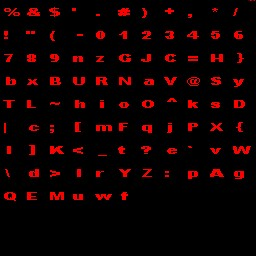This is the check used for checking for the win condition and rendering the flag onto screen.

``````if (hoho == mhm) {
startx = 0.03f;
starty = .35;
deltax = .029;
deltay = .06;
if (UV.x > startx && UV.x < startx + 32. * deltax && UV.y > starty && UV.y < starty + deltay) {
int i = int((UV.x - startx) / deltax);
float fi = float(i);
int q = int(d[i] ^ mhm2.x ^ mhm2.y);
int qy = q / 11;
q %= 11;
float cx = tux * float(q) + (UV.x - (startx + deltax * float(fi))) * tux / deltax;
float cy = tuy * float(qy) + ((UV.y - starty) * tuy / deltay);
float r = texture(tex2, vec2(cx, cy)).r;
color = mix(vec4(r) * vec4(sin(tock*.1 + uv.y), cos(tock*3 + uv.x), .0, 1.), color, 1. - r);
}
deltax = 0.025;
deltay = 0.05;
}
``````

The snippet computes the index in the text atlas with int(d[i] ^ mhm2.x ^ mhm2.y and then renders it.

In fact we can edit the shaders in RenderDoc to see what happens. Let's change the if condition to always evaluate to true and modify the index computation to be int((d[i] ^ mhm2.x ^ mhm2.y) & 0xFF) and then we get: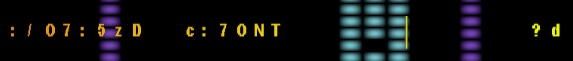It's some broken text but at least we learn that we should get the flag printed in the middle when we reach a win condition. The win value is contained in the mhm uniform and its value is set to 1337. So, the player has to get a score of 1337 to get the flag.

Furthermore, there are the two values mhm2.x and mhm2.y with which the index is xor-ed. Inspecting the values does not reveal anything but they seem to be some large xor masks, correspondingly 0x71272A0A and 0x60ABD0BD. The two values will be relevant later.

Also, the d[] array being accesses is the same as the one to which it is written in the compute shader. This reveals the whole way the flag is generated. Each time the player scores, the d[] array gets updated. Once a score of 1337 is reached, the fragment shader computes the indices in the text atlas using the d[] array and the two values in the mhm2 vector.

We can try to get the flag by again modifying the shader to reach this condition. First we remove the early return in the compute shader even if the game is over. Then, we rewrite the code for incrementing the score and decoding the flag to:

``````for (int i = 0; i < 1337; ++i) {
if (row == 0)
{
if ((col != 0u) && (col != 15u))
{
for (int r = int(row); r < 32; r++)
{
_234.arr2[(r * 16) + int(col)] = _35.arr[((r + 1) * 16) + int(col)];
_35.arr[(r * 16) + int(col)] = _35.arr[((r + 1) * 16) + int(col)];
}
}
if (col == 0u)
{
_299.abra++;
}
}
memoryBarrierShared();
barrier();
if (row == 0)
{
uint q = _299.d[(col + uint(_299.abra)) % 32u];
q ^= (uint(_299.abra) + col);
_299.d[(col + uint(_299.abra)) % 32u] = (q << uint(25)) | (q >> uint(10));
q = _299.d[((col + uint(_299.abra)) + 16u) % 32u];
q ^= ((uint(_299.abra) + col) + 16u);
_299.d[((col + uint(_299.abra)) + 16u) % 32u] = (q << uint(25)) | (q >> uint(10));
}
memoryBarrierShared();
barrier();
}
``````

We save by clicking F5 and then re-run the captured frame by clicking Tools -> Start Replay Loop. And then we get a broken flag again:It looks like there might be something else happening, so we have to examine the binary.

### Assembly debugger

If we open the executable in pebear, then we can see that there is only one section named peippo and that the only two imports are LoadLibraryA and GetProcAddress. That's not very helpful.

By opening it in x32dbg we can examine it and observe that in fact the game is packed using an unknown custom packer. The CFG is the following:If we just set a breakpoint on the last jmp instruction and reach it, we can see that the graph was altered after unpacking: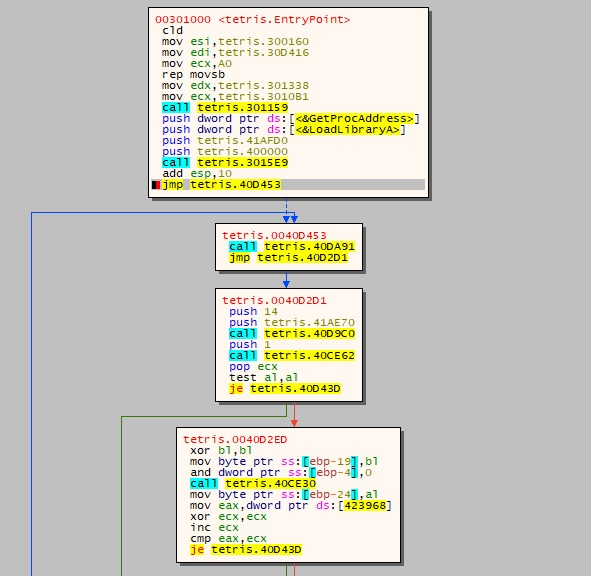I find it useful to step-through the execution of the program in the CFG. Eventually we reach our first anti-debugger check which reads the PEB::BeingDebugged byte and sets the result at address 0x424FD4.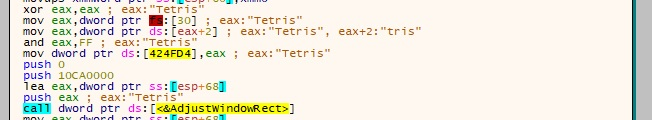We can continue stepping-through and we reach another anti-debugger check which queries the running processes, computes a hash of their names and compares them against known hashes.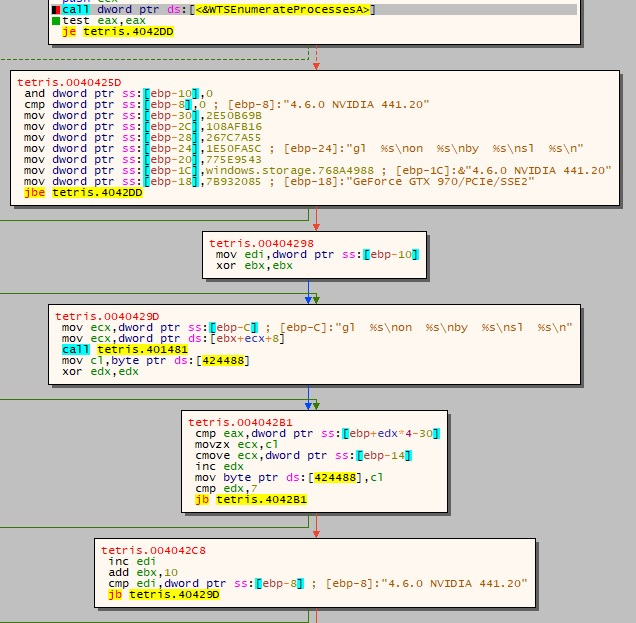This anti-debug check is bad since it can give false positives and also makes the game unwinnable if the player happens to have processes which happen to collide with the hashes. but it's easy to verify that some debuggers like OllyDbg, x32dbg and RenderDoc are detected via it in a sneaky way without making any strings present. The check sets the value at 0x424488 to 1 if a debugger process is running.

After setting "Hardware break points" on the addresses 0x424488 and 0x424FD4, we eventually reach the places where the values are used.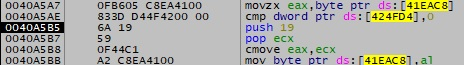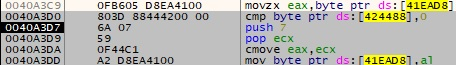One of the values is set if no debugger is attached and the other one if no debugger process is running.

To find out the intention of setting these bytes only under these conditions we have to continue stepping through the program. If we continue examining resources, we can see the text atlas being unpacked and loaded into a GL texture but that's not where the bytes are being written.

Let's examine the shaders and shader binary which are used. Unfortunately, since the exported GL functions depend on context initialization, the driver does not statically export them and thus we cannot find it using x32dbg at least in the list of exported functions in the nvogl32.dll (NVIDIA user-space GL driver). But we can search for the function strings which are passed to wglGetProcAddress and set hardware break points. Eventually we find out that the written bytes above are targetting the SPIR-V binary.

We can run the program twice: once with debugger attached and running, and once with both values unset by us. Then we can dump both SPIR-V binaries, decompile them using any of the Khronos tools and compare them:

``````266c266
<         _299.d[(col + uint(_299.abra)) % 32u] = (q << uint(13)) | (q >> uint(10));
---
>         _299.d[(col + uint(_299.abra)) % 32u] = (q << uint(25)) | (q >> uint(7));
269c269
<         _299.d[((col + uint(_299.abra)) + 16u) % 32u] = (q << uint(13)) | (q >> uint(10));
---
>         _299.d[((col + uint(_299.abra)) + 16u) % 32u] = (q << uint(25)) | (q >> uint(7));
``````

So, so apparently we were working with the unmodified shader in RenderDoc.

Furthermore, the contents of the fragment shader and the spirv binary are used to compute the two hash values with which the text atlas indices are xor-ed when displaying the flag: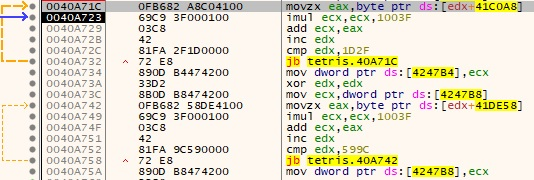The two values with the anti-debugger checks disabled are: 0x71272A0A and 0x515ECC80.

We can continue investigating and notice that we enter the game loop and that there are no more sneaky checks any longer. With this we can move to the actual solution.

### Dynamic solution in RenderDoc

A dynamic solution in RenderDoc would be easy since the debugger provides us with an easy interface for editing shaders and reloading them from a fixed state.

First we modify the compute shader to increase the score 1337 times and to apply each iteration of the rotation hash:

``````for (int i = 0; i < 1337; ++i) {
if (row == 0)
{
if ((col != 0u) && (col != 15u))
{
for (int r = int(row); r < 32; r++)
{
_234.arr2[(r * 16) + int(col)] = _35.arr[((r + 1) * 16) + int(col)];
_35.arr[(r * 16) + int(col)] = _35.arr[((r + 1) * 16) + int(col)];
}
}
if (col == 0u)
{
_299.abra++;
}
}
memoryBarrierShared();
barrier();
if (row == 0)
{
uint q = _299.d[(col + uint(_299.abra)) % 32u];
q ^= (uint(_299.abra) + col);
_299.d[(col + uint(_299.abra)) % 32u] = (q << uint(25)) | (q >> uint(7));
q = _299.d[((col + uint(_299.abra)) + 16u) % 32u];
q ^= ((uint(_299.abra) + col) + 16u);
_299.d[((col + uint(_299.abra)) + 16u) % 32u] = (q << uint(25)) | (q >> uint(7));
}
memoryBarrierShared();
barrier();
}
``````

Next we edit the fragment shader to use the correct shader hashes:

``````if (hoho == mhm) {
startx = 0.03f;
starty = .35;
deltax = .029;
deltay = .06;
if (UV.x > startx && UV.x < startx + 32. * deltax && UV.y > starty && UV.y < starty + deltay) {
int i = int((UV.x - startx) / deltax);
float fi = float(i);
int q = int(d[i] ^ 0x71272A0A ^ 0x515ECC80);
int qy = q / 11;
q %= 11;
float cx = tux * float(q) + (UV.x - (startx + deltax * float(fi))) * tux / deltax;
float cy = tuy * float(qy) + ((UV.y - starty) * tuy / deltay);
float r = texture(tex2, vec2(cx, cy)).r;
color = mix(vec4(r), color, 1. - r);
}
deltax = 0.025;
deltay = 0.05;
}
``````

Remember to click the Refresh button in RenderDoc for both shaders, then go to Tools -> Start replay loop and there you go: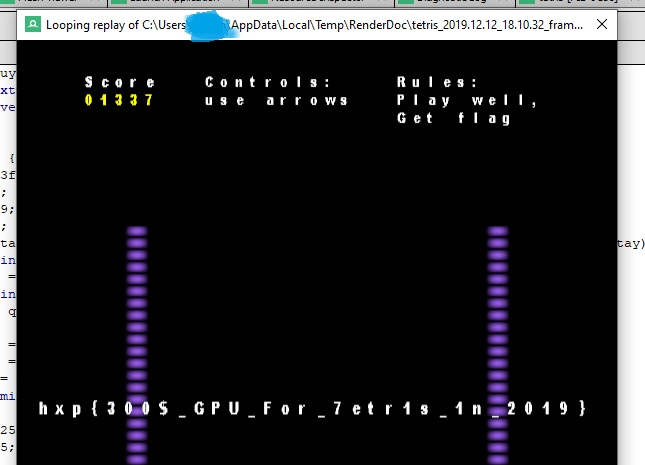### A static solution

Solving the challenge is also possible without a graphics debugger but it requires one to extract the text atlas, shaders and spirv binary. All of that can be done with the earlier steps but it might be a bit more difficult to analyze and experiment with.

Decompiling the SPIR-V binary can be done with SPIRV-Cross which outputs a GLSL shader. In fact RenderDoc uses that tool internally to decompile the SPIR-V binary.

After extracting all of the shaders and GPU buffers, one can write a decoder:

``````h1=0x71272a0a
h2=0x515ecc80
bt = [
0x34EDC49F, 0x16ACC9CF, 0x705071FF, 0x5370F9EF,
0x14BAC19F, 0x36F8C9DB, 0x50357BEB, 0x726F73FB,
0x85348B0A, 0xE746831F, 0x81BA198F, 0xA3C8419F,
0x4C8FF9EF, 0x3ACD71FF, 0x5C3D69E5, 0x7E48F4F5,
0xA7FB8D06, 0x80CB0516, 0x46331D24, 0x64769464,
0x8BA38414, 0xA9958C04, 0xE5499434, 0xC7309C31,
0x3AE76E40, 0x18BF2650, 0x7CD73E60, 0x16BF3671,
0xF9678EA5, 0xDB24D4B5, 0xBDD94C85, 0x8B01C495]

target=1337
for u in range(target):
for v in range(32):
q = (u+1+v) ^ bt[(u+1+v)%32]
bt[(u+1+v)%32] = ((q<<25)&0xFFFFFFFF) | (q>>7)
for u in range(32):
bt[u] = bt[u] ^ h1 ^ h2

text_map = {}
text_map[(6,3)] = "h"
text_map[(7,1)] = "x"
text_map[(8,3)] = "n"
text_map[(5,10)] = "{"
text_map[(9,7)] = "3"
text_map[(9,4)] = "0"
text_map[(3,8)] = "p"
text_map[(10,2)] = "\$"
text_map[(4,4)] = "_"
text_map[(8,5)] = "G"
text_map[(5,8)] = "P"
text_map[(7,3)] = "U"
text_map[(5,5)] = "F"
text_map[(6,5)] = "o"
text_map[(3,4)] = "r"
text_map[(8,0)] = "7"
text_map[(4,7)] = "e"
text_map[(4,5)] = "t"
text_map[(9,5)] = "1"
text_map[(6,9)] = "s"
text_map[(9,6)] = "2"
text_map[(8,2)] = "9"
text_map[(8,10)] = "}"

flag = ""
for u in range(32):
row = int(bt[u] / 11)
col = bt[u] % 11
if (row, col) not in text_map:
flag = flag + "?"
else:
flag += text_map[(row, col)]
print(flag)
``````

Note that the text_map dictionary has entries only for what's queried from the text atlas.

The script prints out the flag: hxp{300\$_GPU_For_7etr1s_1n_2019}

### The story behind the challenge

At some point during the CTF preparation there was a shortage of RE challenges, so after a bump into kirschju we agreed that I can contribute with something small. The challenge was really supposed to be a filler for the RE category and be developed using whatever tools I had left from my demoscene days.

The initial version was built was on top of the Macau Exports 64k tool and used my packer to get the binary small and also thwart static analysis and patching without at least running it. Eventually I switched to another framework for creating the challenge since the original 64k tool was going at great lengths to keep the binary small and the code base was spaghetti.

We ran into multiple issues during the development phase:

• For some reason the game does not run on AMD when the SPIR-V binary is used instead of plain GLSL.
• For some reasons GLSL's atomicMin does not work on Intel and the Intel compiler does not complain. Maybe it's an issue coming from the SPIR-V translation. The fix was to rewrite the code to use a min-reduction.
• The NVIDIA user-space driver used to corrupt its heap once glSpecializeShader was called. That could be a bug coming from me.

I hope the challenge was enjoyable and did not cause much frustration for those who were not able to run it.